-=+=- -=+=- -=+=- -=+=- -=+=- -=+=- -=+=- -=+=- -=+=- -=+=- -=+=- -=+=- -=+=- -=+=- -=+=- -=+=- -=+=- -=+=- -=+=- -=+=- -=+=- -=+=- -=+=- -=+=- -=+=- -=+=- -=+=- -=+=- -=+=- -=+=- (c) WidthPadding Industries 1987 0|519|0 -=+=- -=+=- -=+=- -=+=- -=+=- -=+=- -=+=- -=+=- -=+=- -=+=- -=+=- -=+=- -=+=- -=+=- -=+=- -=+=- -=+=- -=+=- -=+=- -=+=- -=+=- -=+=- -=+=- -=+=- -=+=- -=+=- -=+=- -=+=- -=+=- -=+=-

Pakz# Orientation of point vs line

24th November 2019

Is a point left or center or right of a line

Pakz# Convex Hull or Gift Wrapping algorithm

21st November 2019

create lines around set of points.

Pakz# Polygon vs Polygon Collision

13th November 2019

Is one polygon colliding with another polygon

Pakz# Point versus Polygon Collision

2nd November 2019

Check if a point is inside a polygon

Pakz# Shortest turn angle vs target angle

27th October 2019

Get distance between angle and target angle. (turrets/homing missiles)

Pakz# Random Bag

16th July 2019

Get more predictable numbers

Pakz# Rivers on a top down map.

30th May 2019

Create 2d Rivers

Pakz6th April 2019Create a greyscale stone like texture

Pakz# Bitwise technique

23rd January 2019

Id System for neighbouring tiles

Pakz# Maps or oilish paintings

21st January 2019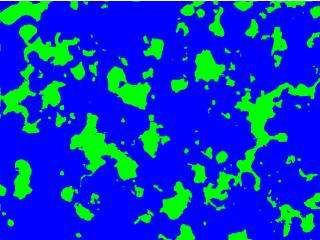Smearing a additive brush

Pakz# Pixel Art Pine Tree(s)

21st January 2019Generate sheets of pine trees(xmas?)

Pakz# Seamless Grow Tiles(grass/desert etc)

2nd July 2018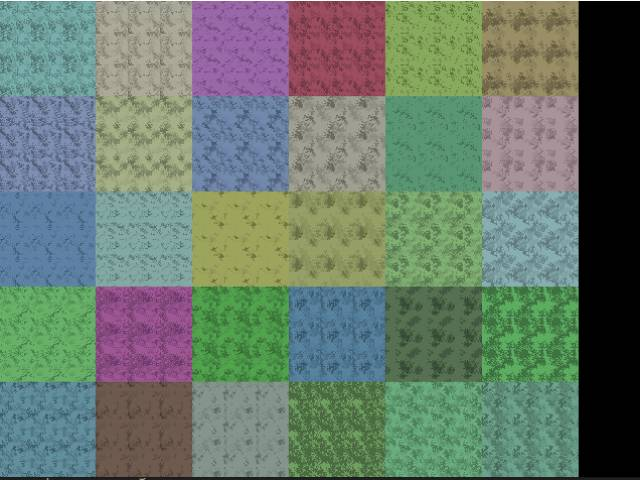Create Tiles with zones method

Pakz# Maps - Horizontal Line Puller

20th June 2018Pull sections from a line to create maps

Pakz# Map Generator Lines and Zones(grow)

20th June 2018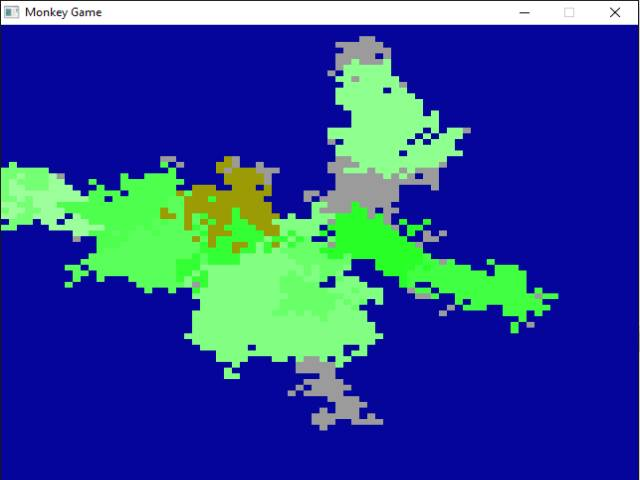2d Maps

Pakz# Chunk System example (2d)

23rd April 2018

Basic Chunks system example.

Pakz# 2d Metaballs

4th February 2018

Old Demoscene effect

Pakz# Horizontal Hall with Rooms Generator

28th January 2018Hall with Rooms generator

Pakz# Brute Force Pathfinding

6th January 2018

Create a path by doing all combinations(grow number)

Pakz# 10 Print Chr (maze)

12th November 2017

Commodore 64 One line Maze Generator

Pakz# Lerp function (Linear Interpolation)

12th November 2017

Return number between low and high

Pakz# Zelda'ish Fantasy map generator

4th November 2017Generate 2d fantasy maps

Pakz23rd October 2017Create Bottle and/or flask like images

Pakz30th August 2017

Function+example that adds binary up by 1

Pakz# Cyclic Map Generator

30th August 2017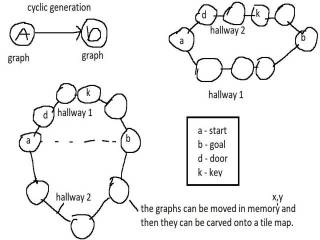Generate maps where you travel/explore in a circle

Pakz# Diffusion Limited Aggregation Maps

1st August 2017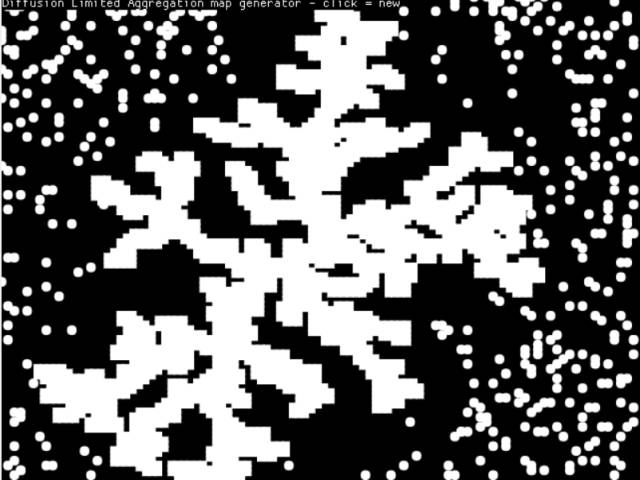Coding challenge from the coding train

More - Older Posts